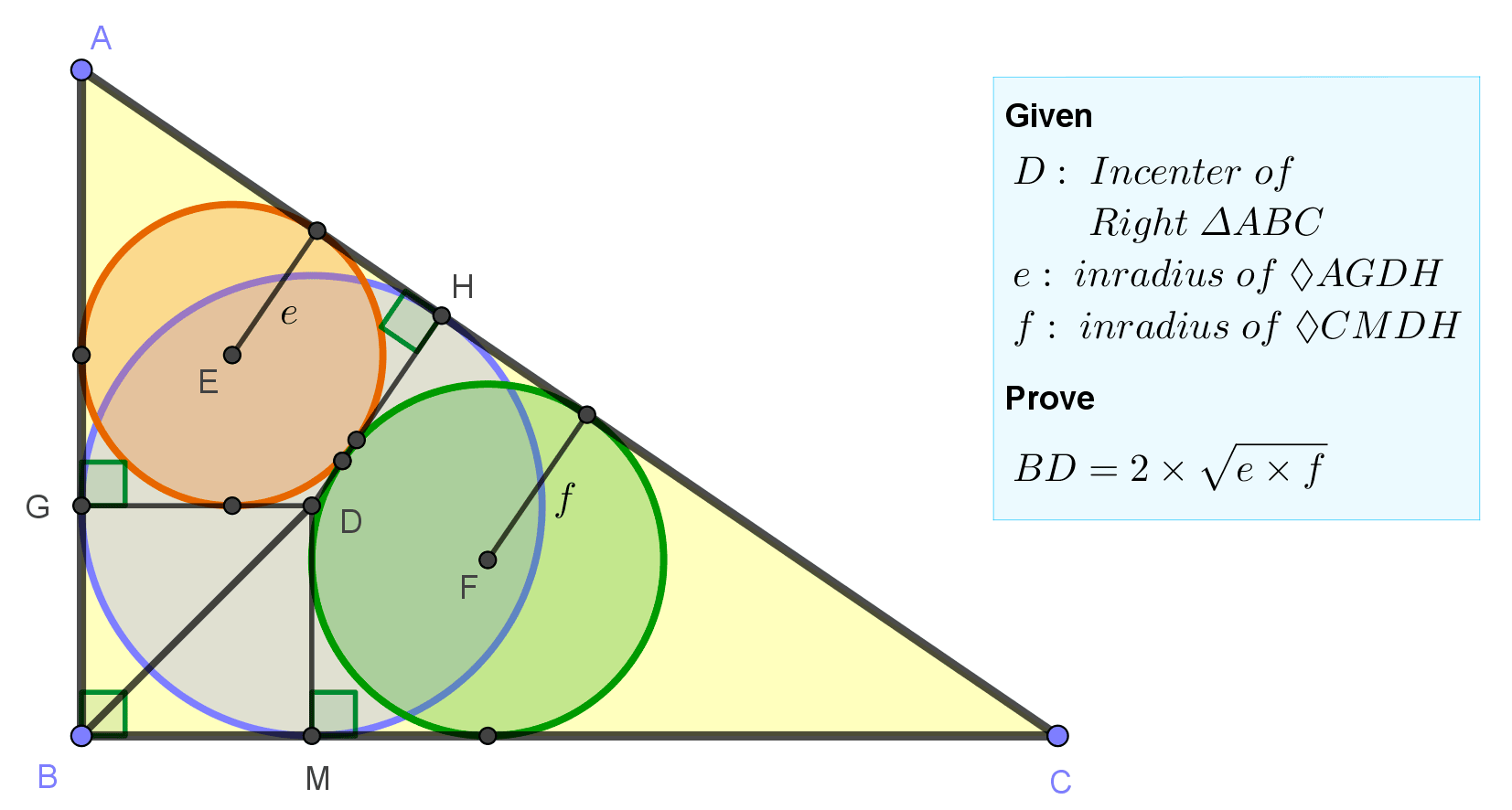# Geometry Problem 1502: The Geometric Intricacies of a Right Triangle's Incircle, Inradii and Tangential Quadrilateral

In the figure, D is the incenter of right triangle ABC, $$e$$ and $$f$$ are the inradii of the tangential quadrilaterals AGDH and CMDH, respectively. Prove that $$BD=2\cdot\sqrt {e\cdot f}$$## Definitions

• Incircle is a circle inscribed within another shape touching every side of it at one point.
• Incenter is the center point of an incircle.
• Tangential quadrilateral is a convex quadrilateral whose sides all can be tangent to an incircle